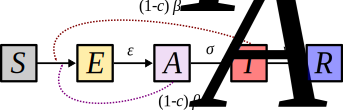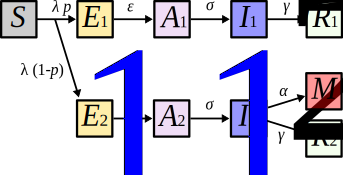# Disclaimer

This text is for research and educational purposes only not a decision-making advice. The following calculations are made using voluntarily simplified assumptions.

For any question, please refer to the French public health authority, Santé Publique France, where one can find all official instructions: https://www.gouvernement.fr/info-coronavirus

The World Health Organisation (WHO) provides as well a comprehensive website: https://www.who.int/fr/emergencies/diseases/novel-coronavirus-2019

# Context

On March 11th, 2020, the WHO announced that the COVID-19 outbreak had reached pandemic stage. On March 12th, the French authorities announced the closure of school structures starting from March 16th. On March 14th, the Prime Minister announced the transition to the third stage of the fight plan against the epidemic and the closure of most of the country’s infrastructure.

Several research groups published more or less detailed versions of mathematical models of the spread of the epidemic online. The structure of the deterministic mathematical model described below includes aspects of some of these models. The internet sites (most of them in English) are referenced at the end of the note.

This model is more complex than the classical (SIR) model, but its structure remains very simple. Indeed, all individuals are assumed to react on average in the same way to infection (there are no differences in age, sex, contacts). Moreover, there is no spatial structure in the model where everyone is potentially in contact with everyone. In summary, at best this model captures the dynamics of the epidemic in a city in France with limited differences across ages.

In this note, we present the model and its hypotheses. Then we calculate the expression of the basic reproduction number ($$R_0$$) as a function of the model parameters. Finally, we perform intervention simulations using default parameter values and compare an epidemic suppression strategy (intense but short control) with a mitigation strategy (lighter but sustainable control).

# Life cycle of the infection

## Simple model

COVID-19 is a respiratory disease that is transmitted both directly (from person to person) and indirectly (by viruses present in the environment shed by infected people). The latter route of transmission is neglected here. Susceptible people (noted $$S$$) who are infected go through a stage where they are infected but they are neither infectious nor symptomatic (noted $$E$$ for exposed). Then they become infectious while remaining asymptomatic (noted $$A$$ for asymptomatic). This stage appears to be particularly important in the spread of COVID-19. Symptoms then develop, with the individuals remaining infectious (denoted $$I$$ for infectious). Finally, the infection ends when people are either immune or dead (denoted $$R$$ for removed). This life cycle can be represented using the following flow chart:In order to simulate epidemics, it is necessary to know the rate at which exposed people become infectious ($$\epsilon$$), the rate at which infectious people become symptomatic ($$\sigma$$), the rate at which symptomatic people cease to be infectious ($$\gamma$$), the rate of transmission from asymptomatic people ($$\beta_A$$), and the rate of transmission from symptomatic people ($$\beta_I$$). Finally, parameter $$c$$ corresponds to the intensity of control (percentage of infected people who are confined). On this diagram, we have not represented the arrival of new hosts of type E by migration (at a rate $$\nu$$).

## Model with differential disease severity

Using existing data, this model can be made more complex without adding too many unknown parameters. In practice, we will assume that a fraction $$p$$ of infections are mild and a fraction $$1-p$$ are severe and require hospitalization. This translates into the life cycle below where mild cases are indexed with a “1” and severe cases with a “2”.This model requires some additional parameters: $$\alpha$$ is the mortality rate for severe cases, $$\gamma_2$$ is the recovery rate for severe cases (which may differ from that for mild cases, $$\gamma_1$$) and potentially different transmission rates from asymptomatic or mild ($$\beta_1$$) and from severe ($$\beta_2$$) cases, since here we assume that asymptomatic and symptomatic cases of a given severity degree have identical transmission rates. Finally, on the diagram, $$\lambda$$ is the strength of infection, which is the rate at which susceptible individuals become infected. It is defined by the formula \begin{align} \lambda = \left[ \beta_1~(A_1+I_1+A_2) + \beta_2~I_2 \right] (1-c). \end{align} For the sake of clarity we do not show transmission and migration events on the diagram.

(Rather) technical point : some models incorporate the bifurcation between mild and severe cases after either stage $$E$$, or stage $$A$$ or even stage $$I$$. This is problematic because, for very high values of p (the proportion of mild cases), one finds very low transition rates to severe cases. The problem is that this means that these events occur on average later than events with high rates. However, the fact that an event is rare (e.g. dying from COVID-19), does not mean that it occurs very late in the course of the infection. To avoid this issue we made the waiting time depend on an infection event (passage from $$S$$ to $$E$$). Indeed, it is possible that “by chance” a susceptible person is infected very quickly (after one day) or very late (in two months), whereas for a duration of infection this is biologically unrealistic (you don’t heal in 1 day or 2 months). End of the technical parenthesis.

# Calculation of $$R_0$$

One of the strengths of the $$R_0$$ concept is that it can be calculated from the life cycle of the infectious agent. For our model with differential severity of infections, we can thus show using the Next Generation theorem (Diekmann et alii 1990 J. Math. Biol., Hurford et alii 2010 J R Soc Interface) that, if natural mortality is neglected, then \begin{align} R_0 = \frac{\beta_1~S_0}{\sigma} + p~\frac{\beta_1~S_0}{\gamma_1} + (1 - p)~\frac{\beta_2~S_0}{\alpha + \gamma_2} . \end{align}

The three terms of this expression have an intuitive interpretation because they correspond to a fraction of the secondary infections generated by an infected person during the course of his or her infection (which is the definition of $$R_0$$). The first term corresponds to infections caused by asymptomatic people $$A_1$$ and $$A_2$$ (in fact, $$1/\sigma$$ is the average time spent in the asymptomatic state). The second term corresponds to infections caused by symptomatic mild infections $$I_1$$ (the duration of this phase can be found through $$1/\gamma_1$$ as well as the proportion of mild cases $$p$$). Finally, the last term corresponds to secondary infections caused by severe and symptomatic cases $$I_2$$.

With this expression, we can see that there are several ways to lower $$R_0$$ and thus control the epidemic. For example, the number of susceptible people and the rates of transmission can be reduced (by confining the population, reducing contacts and wearing masks). One can also increase the recovery rates by isolating symptomatic people (from the point of view of the virus, a person who no longer has contacts is equivalent to a recovery, since he or she no longer transmits the virus).

# Parameter ranges

The following table lists the model parameters, the default values chosen and the biological ranges listed. These ranges are often wide due to large variations from one infection to another. For more details, please refer to the MIDAS consortium compilations.

Notation Description Default Range Reference
$$\epsilon$$ rate of end of latency $$1/4.2\ \text{jours}^{-1}$$ $$[0.21\ ;\ 0.27]$$ Li et alii (2020, NEJM)
$$\sigma$$ rate of symptoms onset $$1\ \text{jour}^{-1}$$ $$[0.9\ ;\ 1.1]$$ Ferguson et alii (2020, Rapport 8)
$$\gamma_1$$ recovery rate of mild cases $$1/17\ \text{jours}^{-1}$$ $$[0.025\ ;\ 0.1]$$ Zhou et alii (2020, Lancet)
$$\gamma_2$$ recovery rate of severe cases $$1/20\ \text{jours}^{-1}$$ $$[0.039\ ;\ 0.13]$$ Zhou et alii (2020, Lancet)
$$R_0$$ basic reproduction number $$2.5$$ $$[2\ ;\ 3]$$ our report
$$p$$ proportion of infections that do not require hospitalization $$0.9$$ $$[0.85\ ;\ 0.95]$$ Santé Publique France
$$\theta$$ case fatality ratio of hospital patients $$0.15$$ $$[0.135\ ;\ 0.165]$$ Santé Publique France
$$\alpha$$ mortality rate of severe infections calculé see the formula
$$\nu$$ migration rate $$10^{-6}\ \text{jour}^{-1}$$ ad hoc
$$b$$ decrease in transmission due to hospitalization $$0.2$$ ad hoc
$$c$$ fraction of the $$R_0$$ decreased by public health policies variable

Note that the mortality rate $$\alpha$$ can be expressed as a function of the case-fatality rate of severe cases through the formula \begin{align} \alpha & =\gamma_2~\frac{\theta}{1-\theta}. \end{align}

In addition, the transmission rate $$\beta$$ is parameterized indirectly using the values of $$R_0$$ (between 2 and 3 depending on the country, with values close to 2.5 for France). Reworking the previous equation, we find that \begin{align} \beta & = \frac{R_0}{S_0} \gamma_1 \sigma \frac{\alpha + \gamma_2}{(\gamma_1+p \sigma)(\alpha + \gamma_2) + b \gamma_1 \sigma (1- p)} . \end{align}

# Group immunity and epidemic size

As a reminder, as mentioned in a previous report, the percentage $$P^\star$$ of the population that needs to be immunized (i.e. in compartment $$R$$) in order to prevent a future epidemic is: \begin{align*} P^\star & = 1 - \frac{1}{R_0}. \end{align*}

Furthermore, in the absence of any control measures, the total fraction of the population $$Q^\star$$ that will be affected by the epidemic is found by solving the equation \begin{align*} Q^\star~R_0 + \log(1-Q^\star) & = 0. \end{align*}

# Numerical simulations

In these simulations, we normalized the total population size and represent the percentage of the population infected. This is voluntary because, as noted above, this model has no structure and therefore cannot be applied directly to an entire country but rather to a small town or village.

## Epidemic wave without control policy

In the absence of any control, the epidemic is growing exponentially over time. On the following graph, each colour corresponds to one of the life cycle categories. The grey dashed line corresponds to the fraction of the population that can be accommodated in resuscitation or intensive care (approximately 12,000 beds in total, which means $$1.79E-4$$ per inhabitant).